# Review — A Blur Classification Approach Using Deep Convolution Neural Network (Blur Classification)

## Blur Classification for Hand Gesture Images Using CNN

In this story, A Blur Classification Approach Using Deep Convolution Neural Network, (Tiwari IJISMD’20), by University of Petroleum and Energy Studies, is reviewed. In this paper:

• Four blur categories: motion, defocus, Gaussian, and box blur are classified for hand gesture images by using CNN.
• It is essential to determine the exact blur type for blind image restoration.

This is a paper in 2020 IJISMD. (Sik-Ho Tsang @ Medium)

# Outline

1. Blur Models
2. Blur Classification Framework
3. Experimental Results

# 1. Blur Models

## 1.1. Motion Blur

• The motion blur appears when the object to be captured moves relative to the imaging device:
• where L is the blur length of uniform motion blur.

## 1.2. Defocus Blur

• Images that are captured due to a deprived convergence of light from an object on the sensor plane results in defocus blur (or out-of-focus):
• where R is the circular disk of radius.

## 1.3. Gaussian Blur

• Blur function are usually approximated by the Gaussian function for a large range of devices like optical image camera, microscope, telescope, etc:
• where σ is the Gaussian blur function with a standard deviation.
• Sometimes, this blur is also used to model defocus blur.

## 1.4. Box Blur

• Box blur is a mean filter where each pixel in the output image has mean of its neighbouring pixels defined in some specific region in the input image.
• Most existing methods model the blur as a box blur, which is caused by constant velocity movement:
• where Suv is the set of points in rectangular blur kernel and m*n is the kernel size.

# 2. Blur Classification Framework

## 2.1. Preprocessing & Fourier Transformation & Cropping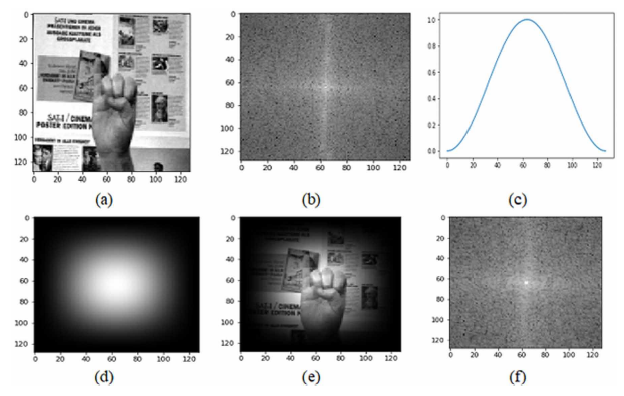(a) Original image, (b) Fourier spectrum of (a), (c) 1-D Hann Window, (d) 2-D Hann window (e) Hann windowed image, (f) Fourier spectrum of (e)
• The periodic boundary conditions and sharp discontinuities among the borders of an image make a cross patterned in frequency spectrum.
• The Haan Window is used to avoid this problem.
• Then, Fourier transformation is applied.
• And the image is 32×32 cropped.

## 2.2. Multi-Layer Perceptron Model (MLPM)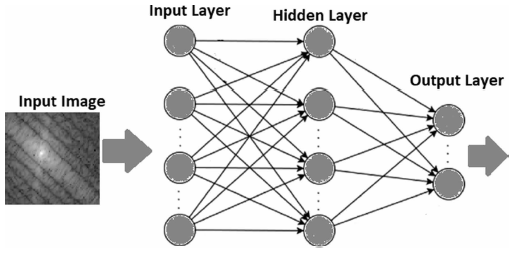A multi-layer perceptron neural network model
• A MLP with just three layers namely input, hidden and output is used.
• The neurons in the first layer i.e. input layer are 1024 and output layer are four, as the input is the 32 × 32 image and the output is a 4 × 1 vector indicating the four blur classes.
• The 500 neurons in the hidden layer are considered.

## 2.3. Convolution Neural Network (CNN)

• In the passage. authors mentioned the CNN model used for blur classification has three convolutional filter’s layers: 64–3×3, 128–3×3, 512–3×3 convolution filters. (But in the figure, only 2 convolutions.)
• 2×2 max pooling is used.
• The last layer is a dense layer that has a softmax activation function with 4 units.
• Dropout is used to reduce overfitting.

# 3. Experimental Results

## 3.1. Datasets

• Triesch gesture database consists of 720 images taken by 24 different persons in 3 dissimilar backgrounds comprise of 10 hand gestures for each, is used.
• All the images are blurred separately using each class of blur namely motion, defocus, box and Gaussian blur. Therefore, the blurred image database has the size of 2880 images.

## 3.2. MLP Results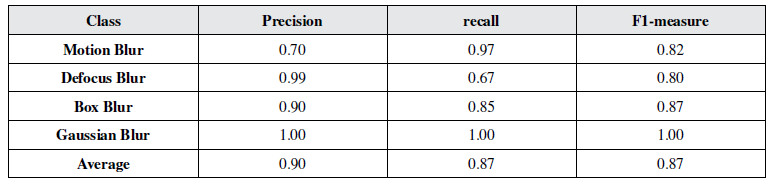Performance with multi-layer perceptron-based classification model
• The blurred image dataset is split into training and validation sets.
• 70% and 30% blurred images are utilized for training and validation separately.
• Whole image dataset is used as test set for the trained model.
• (Whole dataset which overlapped with the training set is strange.)
• The overall test loss and the test accuracy of the model are 0.22 and 0.93, respectively.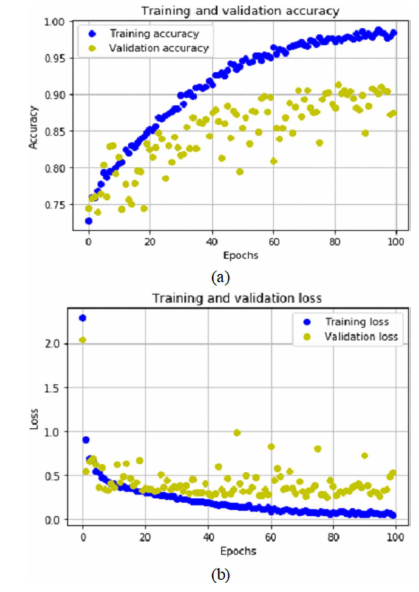The accuracy curves and loss curves separately for training and validation data.

## 3.3. CNN Results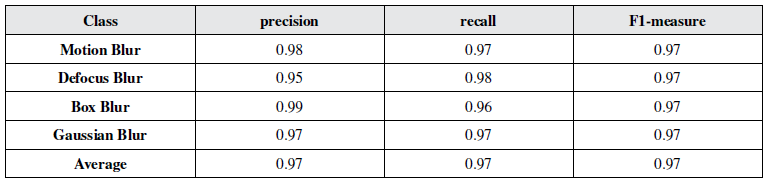Performance with convolution neural network-based classification model
• The dataset split is the same as the MLP one.
• The overall test loss and the test accuracy of the model are 0.10 and 0.97, respectively.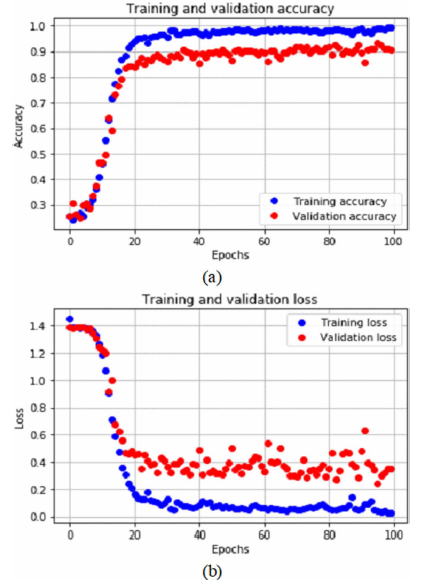The accuracy curves and loss curves separately for training and validation data.

## 3.4. ROC Results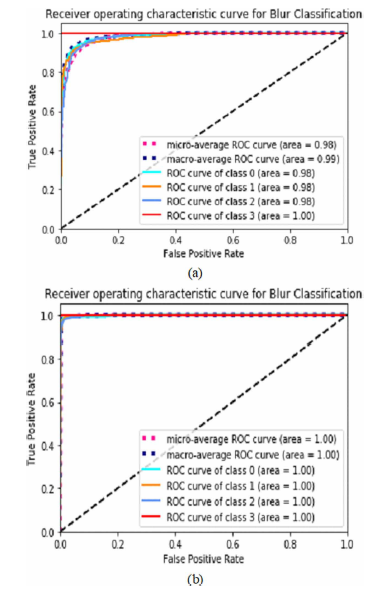(a) ROC Plots for MLP model, (b) ROC Plots for CNN model
• The above shows the ROC plots.

## 3.5. Accuracy and SOTA Comparison

• The average accuracies achieved through MLP and CNN models are 93.0 and 97.0, respectively.
• The synthesis database is also tested with Curvelet transform based energy features and a feed forward neural network-based classification model (Tiwari, 2017). The average accuracy using this model is 95.7.

## Reference

[2020 IJISMD] [Tiwari IJISMD’20]
A Blur Classification Approach Using Deep Convolution Neural Network

## Blur Classification

2017 [SFA] 2019 [SFA & SFGN] 2020 [Szandała SSCI’20] [Tiwari IJISMD’20]

--

--